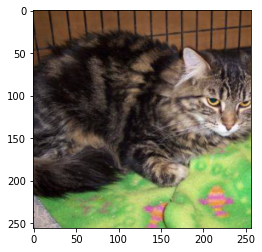### How to calculate mean and standard deviation of images in PyTorch

• Calculate the mean and standard deviation of the image dataset.
First, we load our images/ image dataset. To load a custom image dataset, use torchvision.datasets.ImageFolder()

The images are arranged in the following way:

`root/class_1/xxx.png root/class_1/xxy.png root/class_1/[...]/xxz.png example: dataset/cat/101.png dataset/cat/1002.png dataset/cat/[...]/1000.png`

In our dataset, we have many cat images as above mentioned in the example.

`# python code to load the image datasetimport torchvisionfrom torchvision import transformsfrom torch.utils.data import DataLoaderdata_path = './dataset/'transform_img = transforms.Compose([    transforms.Resize(256),    transforms.CenterCrop(256),    transforms.ToTensor(),])image_data = torchvision.datasets.ImageFolder(  root=data_path, transform=transform_img)image_data_loader = DataLoader(  image_data,   batch_size=len(image_data),   shuffle=False,   num_workers=0)`

Visualize an image from the image dataset.

`Python3# Python code to visualize an imageimport matplotlib.pyplot as pltimages, labels = next(iter(image_data_loader))def display_image(images):  images_np = images.numpy()  img_plt = images_np.transpose(0,2,3,1)  # display 5th image from dataset  plt.imshow(img_plt)display_image(images)`

Output:#### Calculate the mean and standard deviation of the dataset

When the dataset is small and the batch size is the whole dataset. Below is an easy way to calculate when we equate batch size to the whole dataset.

`Python3# python code calculate mean and stdfrom torch.utils.data import DataLoaderimage_data_loader = DataLoader(  image_data,   # batch size is whole datset  batch_size=len(image_data),   shuffle=False,   num_workers=0)def mean_std(loader):  images, lebels = next(iter(loader))  # shape of images = [b,c,w,h]  mean, std = images.mean([0,2,3]), images.std([0,2,3])  return mean, stdmean, std = mean_std(image_data_loader)print("mean and std: \n", mean, std)`

Output:
mean and std:
(tensor([0.5125, 0.4667, 0.4110]), tensor([0.2621, 0.2501, 0.2453]))

If our dataset is large and we divide the dataset into batches we can use the below python code to determine the mean and standard deviation.

`Python3# python code to calculate mean and std import torchfrom torch.utils.data import DataLoaderbatch_size = 2loader = DataLoader(  image_data,   batch_size = batch_size,   num_workers=1)def batch_mean_and_sd(loader):        cnt = 0    fst_moment = torch.empty(3)    snd_moment = torch.empty(3)    for images, _ in loader:        b, c, h, w = images.shape        nb_pixels = b * h * w        sum_ = torch.sum(images, dim=[0, 2, 3])        sum_of_square = torch.sum(images ** 2,                                  dim=[0, 2, 3])        fst_moment = (cnt * fst_moment + sum_) / (cnt + nb_pixels)        snd_moment = (cnt * snd_moment + sum_of_square) / (cnt + nb_pixels)        cnt += nb_pixels    mean, std = fst_moment, torch.sqrt(snd_moment - fst_moment ** 2)            return mean,std  mean, std = batch_mean_and_sd(loader)print("mean and std: \n", mean, std)`
Output:
mean and std:
(tensor([0.5125, 0.4667, 0.4110]), tensor([0.2621, 0.2501, 0.2453])

#### Useful Resources:

You may be interested in the following posts.